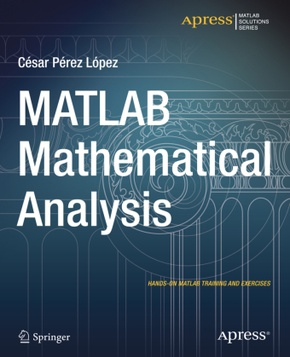# MATLAB Mathematical Analysiszum Preis von:
48,10€
Preis inkl. Mwst. versandkostenfrei
Kartoniert/Broschiert
Springer, Berlin, 2015, 368 Seiten, Format: 19x23,4x1,9 cm, Artikeltyp: Englisches Buch, ISBN-10: 148420350X, EAN: 9781484203507, Bestell-Nr: 48420350UA

### Reduzierte Artikel in dieser Kategorie

Produktbeschreibung

MATLAB Mathematical Analysis is a reference book that presents the techniques of mathematical analysis through examples and exercises resolved with MATLAB software. The purpose is to give you examples of the mathematical analysis functions offered by MATLAB so that you can use them in your daily work regardless of the application. The book supposes proper training in the mathematics and so presents the basic knowledge required to be able to use MATLAB for calculational or symbolic solutions to your problems for a vast amount of MATLAB functions.

The book begins by introducing the reader to the use of numbers, operators, variables and functions in the MATLAB environment. Then it delves into working with complex variables. A large section is devoted to working with and developing graphical representations of curves, surfaces and volumes. MATLAB functions allow working with two-dimensional and three-dimensional graphics, statistical graphs, curves and surfaces in explicit, implicit, parametric and polar coordinates. Additional work implements twisted curves, surfaces, meshes, contours, volumes and graphical interpolation.

The following part covers limits, functions, continuity and numerical and power series. Then differentiation is addressed in one and several variables including differential theorems for vector fields. Thereafter the topic of integration is handled including improper integrals, definite and indefinite integration, integration in multiple variables and multiple integrals and their applications.

Differential equations are exemplified in detail, Laplace transforms, Tayor series, and the Runga-Kutta method and partial differential equations.

#### Inhaltsverzeichnis:

Chapter 1: MATLAB Introduction and Working Environment

Chapter 2: Numbers, Operators, Variables, and Functions

Chapter 3: Complex Numbers and Complex Variable Functions

Chapter 4: Graphics in MATLAB Curves, Surfaces, and Volumes

Chapter 5: Limits of Successions and Functions, Continuity, and One and Several Variables Systems

Chapter 6: Numerical Series, Power Series, and Developments in Series

Chapter 7: Derivatives in One and Several Variables

Chapter 8: Integration of One and Several Variables and Applications of Developments in Series

Chapter 9: Differential Equations

#### Rezension:

"This book about the MATLAB environment is designed to offer a generous training support to all users interested in complementary information relative to the documentation provided by the software producer. ... The book is recommended to students and researchers working in different areas ... . Educators may also find motivating applications and case studies for lectures or laboratory sessions." (Octavian Pastravanu, zbMATH 1328.65005, 2016)

### Alle Artikel in der Kategorie

Themen
Verwandte ThemenArtikel

zum Preis von
(Preis inkl. Mwst.)